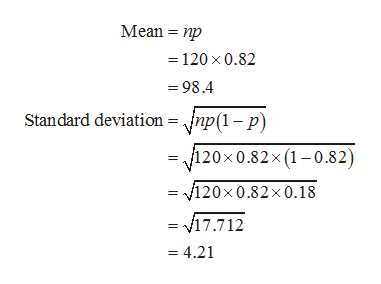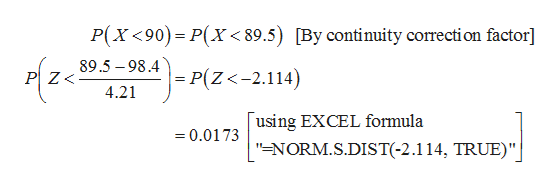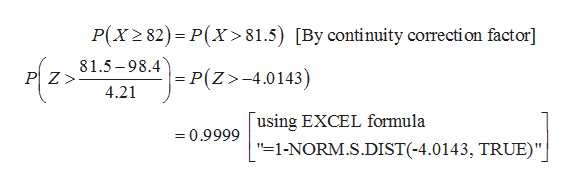# Use the normal approximation to the binominal to approximate the probabilities.A gardener buys a package of seeds. 82% of seeds of this type germinate. The gardener plants 120 seeds.A) what is the probability that fewer than 90 seeds germinate?B) What is the probability that the germinated seeds is more than or equal to 82 seeds?C) What is the probability that the number of seeds that germinate is between 54 and 84, exclusive?

Question
64 views

Use the normal approximation to the binominal to approximate the probabilities.

A gardener buys a package of seeds. 82% of seeds of this type germinate. The gardener plants 120 seeds.

A) what is the probability that fewer than 90 seeds germinate?

B) What is the probability that the germinated seeds is more than or equal to 82 seeds?

C) What is the probability that the number of seeds that germinate is between 54 and 84, exclusive?

check_circle

Step 1

Normal approximation to the binomial:

If a random variable X ~ B(n, p) in which n is large and p is close to 0.5, then the normal distribution can be used as an approximation to binomial distribution.

Computation of Mean and Standard deviation:

Let x be the random variable denotes the number of germination of seeds, which follows binomial distribution.

Here, probability of success, p is 0.82.

Number of seeds, n is 120.

The mean and standard deviation are calculated as follows.help_outlineImage TranscriptioncloseMean np =120 x 0.82 98.4 Standard deviation = ^np(1-p) =120x 0.82x (1-0.82) = 120x0.82x0.18 v17.712 = 4.21 fullscreen
Step 2

A) Computation of probability that fewer than 90 seeds germinate:

The probability that fewer than 90 seeds germinate is P(X < 90) = 0.0173 from the calculations given below.help_outlineImage TranscriptioncloseP(X <90) P(X < 89.5) [By continuity correction factor] 89.5-98.4p(Z<-2.114) PZ< 4.21 using EXCEL formula "NORM.S.DIST(-2.114, TRUE)"| =0.0173 fullscreen
Step 3

B) Computation of probability that the germinated seeds is more than or equal to 82 seeds:

The probability that the germinated seeds is more th...help_outlineImage TranscriptioncloseP(x2 82) P(X>81.5) [By continuity correction factor] 81.5-98.4 P(Z>-4.0143) PZ> 4.21 using EXCEL formula 0.9999 "=1-NORM.S.DIST(-4.0143, TRUE)" fullscreen

### Want to see the full answer?

See Solution

#### Want to see this answer and more?

Solutions are written by subject experts who are available 24/7. Questions are typically answered within 1 hour.*

See Solution
*Response times may vary by subject and question.
Tagged in

### Other